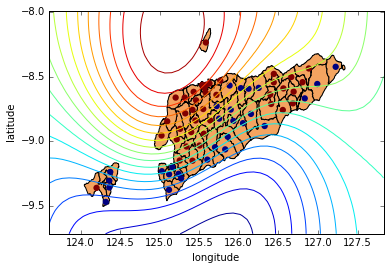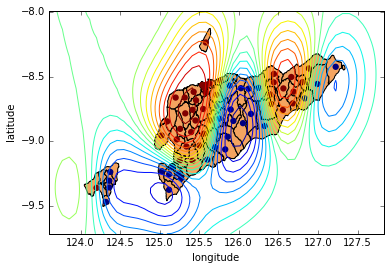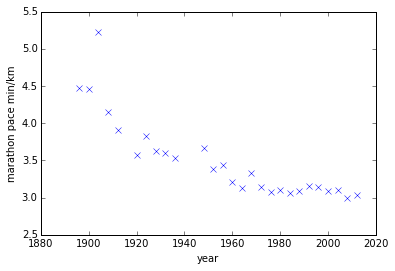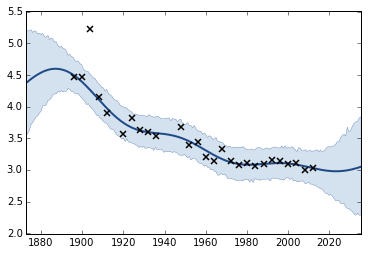# Non Gaussian Likelihoods¶

## Gaussian Winter School, Genova, Italy¶

### Ricardo Andrade Pacheco, Max Zwiessele, James Hensman and Neil Lawrence¶

In this lab we are going to consider approaches to dealing with non-Gaussian likelihoods.

In :
%matplotlib inline
import numpy as np
import matplotlib.pyplot as plt
import GPy
import pods
from IPython.display import display

In :
from matplotlib.patches import Polygon
from matplotlib.collections import PatchCollection
import cPickle as pickle
import urllib

urllib.urlretrieve('http://staffwww.dcs.sheffield.ac.uk/people/M.Zwiessele/gpss/lab2/EastTimor.pickle', 'EastTimor2.pickle')

with open("./EastTimor2.pickle","rb") as f:


Now we will create a map of East Timor and, using GPy, plot the data on top of it. A classification model can be defined in a similar way to the regression model, but now using GPy.models.GPClassification. However, once we've define the model, we also need to update the approximation to the likelihood. This runs the Expectation propagation updates.

In :
print y.shape
print X.shape

(67, 1)
(67, 2)

In :
#Visualize a map of East-Timor
fig = plt.figure()
for p in polygons:
ax.set_xlim(124.,127.5)
ax.set_ylim(-9.6,-8.1)
ax.set_xlabel("longitude")
ax.set_ylabel("latitude")

#Define the model
kern = GPy.kern.RBF(2)
model = GPy.models.GPClassification(X,y, kernel=kern)
display(model)
model.plot(ax=ax)


Model: gp_classification
Log-likelihood: -115.594679675
Number of Parameters: 2

gp_classification. Value Constraint Prior Tied to
rbf.variance 1.0 +ve
rbf.lengthscale 1.0 +ve
Out:
{'contour': <matplotlib.contour.QuadContourSet instance at 0x11104ed88>,
'dataplot': <matplotlib.collections.PathCollection at 0x116438dd0>}The decision boundary should be quite poor! However we haven't optimized the model. Try the following:

In :
model.randomize()
model.optimize('bfgs')

print 'Log likelihood={}'.format(model.log_likelihood())
display(model)

fig = plt.figure()
for p in polygons:
ax.set_xlim(124.,127.5)
ax.set_ylim(-9.6,-8.1)
ax.set_xlabel("longitude")
ax.set_ylabel("latitude")
model.plot(ax=ax)

Log likelihood=-107.199692241


Model: gp_classification
Log-likelihood: -107.199692241
Number of Parameters: 2

gp_classification. Value Constraint Prior Tied to
rbf.variance 1.66833160644 +ve
rbf.lengthscale 0.316603830844 +ve
Out:
{'contour': <matplotlib.contour.QuadContourSet instance at 0x1160957a0>,
'dataplot': <matplotlib.collections.PathCollection at 0x11609d9d0>}The optimization is based on the likelihood approximation that was made after we constructed the model. However, because we've now changed the model parameters the quality of that approximation has now probably deteriorated. To improve the model we should iterate between updating the Expectation propagation approximation and optimizing the model parameters.

## Exercise 1¶

Write a for loop to optimize the model by iterating between EP and kernel parameters optimization. What happens with the decision boundary after these iterations?

In :
# Exercise 1 answer here


### Robust Regression: A Running Example¶

Yesterday we considered the olympic marathon data. In 1904 we noted there was an outlier example. Today we'll see if we can deal with that outlier by considering a non-Gaussian likelihood. Noise sampled from a Student-$t$ density is heavier tailed than that sampled from a Gaussian. However, it cannot be trivially assimilated into the Gaussian process. Below we use the Laplace approximation to incorporate this noise model.

In :
# redownload the marathon data from yesterday and plot
data = pods.datasets.olympic_marathon_men()
X = data['X']
Y = data['Y']

plt.plot(X, Y, 'bx')
plt.xlabel('year')
plt.ylabel('marathon pace min/km')

Out:
<matplotlib.text.Text at 0x1161dad90>In :
# make a student t likelihood with standard parameters
t_distribution = GPy.likelihoods.StudentT(deg_free=5, sigma2=2)
laplace = GPy.inference.latent_function_inference.Laplace()

kern = GPy.kern.RBF(1, lengthscale=20) + GPy.kern.Bias(1)
model = GPy.core.GP(X, Y, kernel=kern, inference_method=laplace, likelihood=t_distribution)
model.constrain_positive('t_noise')

model.optimize()
model.plot()
display(model)

WARNING: reconstraining parameters gp


Model: gp
Log-likelihood: 0.229198900966
Number of Parameters: 5

gp. Value Constraint Prior Tied to# Exercise 2 answer